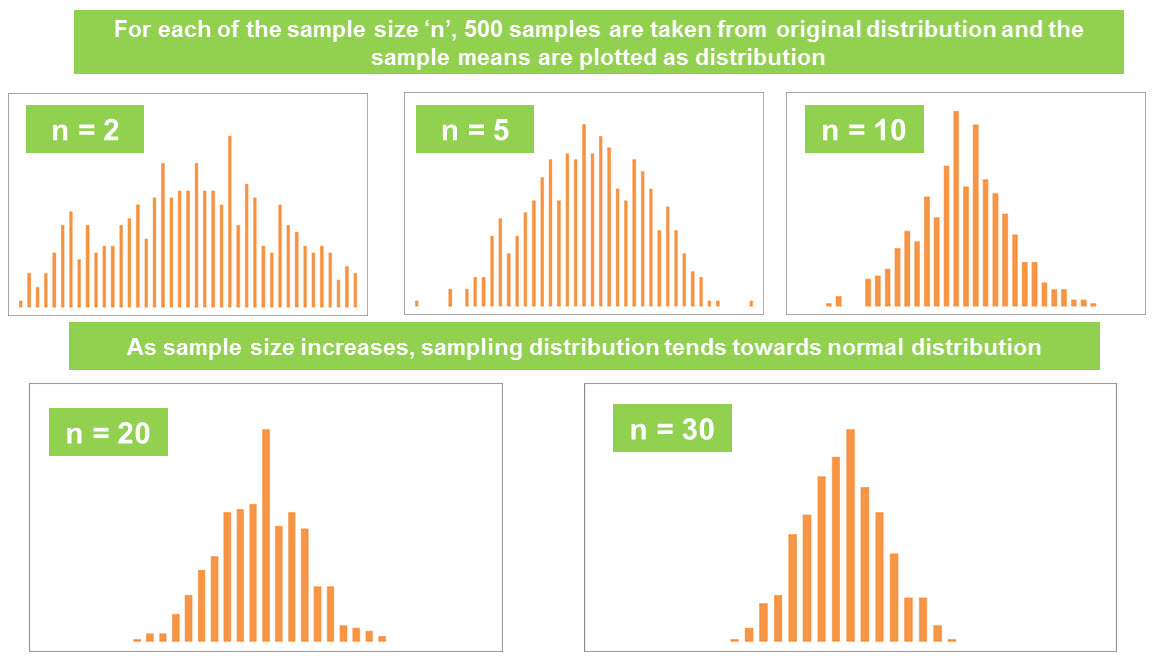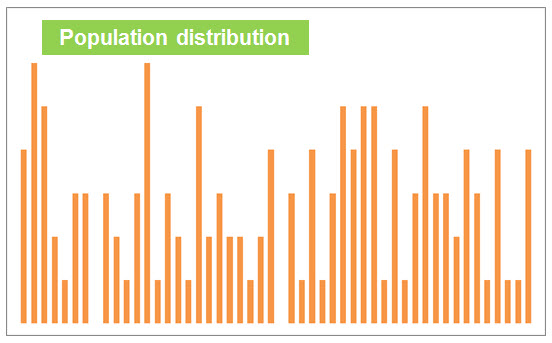Introduction to Central limit theorem

Simply put, it states that the frequency distribution of ‘means or averages’ of samples drawn randomly from a population with finite mean and variance will approximately follow normal distribution provided the sample size is large enough.

Here the most important point is that this is valid regardless of the distribution of parent population from which the sample is drawn (There are few exceptions; Ex: Cauchy distribution)

How to check CLT? (Try out in excel)

• Step 1: Consider any distribution (Need NOT be a Normal one)
• Step 2: Select a sample of size n from distribution in Step 1
• Step 3: Calculate average of above sample (Say A1)
• Step 4:Repeat the process of selecting sample of size n and calculate its mean/average several times (Say  the averages/means are A2, A3, A4….)
• Step 5: Frequency distribution of means of samples which is frequency distribution of A1, A2, A3……
• Step 6: Central limit theorem says that the frequency distribution of means A1, A2, A3…… wil follow an approximate normal distribution if sample size ‘n’ is large enough

I have randomly generated the data for original distribution from which I picked 500 samples for each of the below sample sizes (2, 5, 10, 20 and 30). As you observe in below graphs, ‘distribution of means of samples will approximate to normal distribution as the sample size is large enough’As stated earlier, CLT is true regardless of ‘parent distribution’. Below is the distribution of parent distribution/population distribution I started with.NoteAs the sample size is large enough (>=30), the mean of all the sample means is approximately equal to the mean of the original distribution

Illustration on Central limit theorem

Let us take an example to understand this further. Suppose, we are examining the one year stock returns. There could be few thousands of stocks listed in that exchange (This is called parent population).

Now, let us randomly pick ‘n’ stocks and calculate the average one year return for these stocks. Let us repeat this step of randomly selecting ‘n’ stocks and compute the average one year return.

• Central limit theorem says that the frequency distribution of these ‘average returns’ or ’mean returns’ of randomly drawn samples follow an approximate normal distribution. Here the sample size is ‘n’ stocks.

• Central limit theorem holds true if the sample size ‘n’ is at least 30 (Few statisticians claim this as 40) and regardless of parent population distribution (In this case it is the distribution of one year returns of ALL stocks).

Importance of central limit theorem

Importance of this theorem comes from the fact that this holds true regardless of parent population distribution. In practical world, we may have to deal with distributions that are not usually normal. However, the ‘means’ of randomly drawn samples from this non-normal population will still follow approximate normal distribution.

This is helpful to estimate the characteristics of entire population from the randomly drawn sample (Primary research or surveys) as we can assume that the sample mean follows normal distribution.

What can be done with a normal distribution? What inferences could be made and what insights could be drawn?

Properties of normal distribution

Any normal distribution regardless of the mean and standard deviation will have certain properties described below.

• 68.27% of the total population can be found in the range of Mean – 1*Std devn and Mean + 1* Std devn. (Area under this range is 0.6827)
• 95.45% of the total population can be found in in the range of Mean – 2*Std devn and Mean + 2* Std devn. (Area under this range is 0.9545)
• 99.73% of the total population can be found in in the range of Mean – 3*Std devn and Mean + 3* Std devn. (Area under this range is 0.9973)
• Similarly, we can find the area at various ranges of the value of random variable x.

Area under the normal curve

Area under the normal curve is 1 as it sums up the probability of a random variable. For example, let us consider the distribution of marks in a class. Here the random variable is the value of marks obtained and the values on y axis denote the probability that a student obtains a particular mark.

Sum of all probabilities becomes the area under the normal curve.

Area = P(Student receiving 0 marks) + P(Student receiving 1 mark) + ….. + P(Student                            receiving 100 marks)

Which is equivalent to saying

Area    =    [(# students at 0 marks)/ (Total students in class)] + [(#students at 1 marks)/ (Total students in class) ]+……. [(#students at 100 marks)/ (Total students in class)] = 1

Standard normal curve

Since the properties of any normal distribution remains same, it is better to convert the normal curve at hand to standard normal curve for the ease of making area calculations. Standard normal curve has mean 0 and standard deviation of 1.

The standard normal z tables are easily available and they can be used to calculate the probability that z is greater than a particular value or the probability that it falls in a particular range.Z value in Standard normal distribution

For example, in our marks distribution example (mean is 70 and std devn is 10) we want to calculate the number of students who scored above 80 or the probability a student scores above 80 can be done as shown below.

P(X > 80) = P (z > ((80-70)/10) = P (z > 1).

This probability of Z >1 could be easily found in the Normal distribution tables and areas.